Use Google's Chrome browser for proper viewing.

IB Physics HL - Year 2 (2019-2020)

8/15

• Informational Packet / Syllabus
• Welcome back for another year of IB Physics! You are going to learn a ton as we uncover the rules that govern the universe!
• Homework - prepare for a Socratic Seminar on Chapter 1 in your new booklet

8/19

• Socratic Seminar on what physics can and cannot do, or say
• PowerPoint introduction to electric charge, conductors and insulators, and the triboelectric series

8/21

• balloon lab
• discuss polarization of conductors and insulators; define an electric dipole
• use Coulomb's Law to calculate the electric force between charges
• Homework - complete the problems on this handout; skip #7

8/23

• tape lab
• review homework and practice using Coulomb's Law
• Homework - do problem on board; read Chapter 2 in The Big Picture booklet
• Notice: test over electrostatics next Thursday

8/27

• define the electric field - handout
• explore depictions of the electric field
• explore electric field strength with a Van de Graaff generator (IRL and in the 3D VR simulation below)

8/29

• test over electrostatics
• Homework - read pp. 183-186 in your IB Physics textbook; complete the packet of IB test questions on electric fields, and do the "breakdown of air" problem
Lab Report Format

3D VR simulation of electromagnetic phenomena

(Maroon VR  - website)

Electric Field and Potential Simulation

Unit One-Page Overviews

5.1 Electric Fields

3D Electrostatic Fields Applet

)

PhET Interactive Simulation - Charges and Fields

)

9/3

• review homework and discuss particle motion through electric fields
• calculate the electric field within a parallel plate capacitor
• homework - complete the notes sheet/ handout

9/5

• finish discussion of a cathode ray tube (CRT) - video
• graph the energy (as a function of separation) of a two-particle system
• homework - complete the packet on energy

9/9

• discuss gravitational potential energy and calculate the escape speed, at the surface of the Earth, for an unpowered rocket - handout
• examine this xkcd infographic on gravity wells
• homework - complete the handout; read the NewScientist article, at right

9/11

• test over electric fields and electrical and gravitational potential energy
• homework - review this handout, then answer these questions (answer key)

9/13

• practice solving problems regarding forces, fields and energy (answer key)
• homework - complete the problems from above and do test corrections for points back on the test

9/17

• discuss the concept of electric potential - notes and problems
• explore equipotentials using the Electric Field and Potential Simulation, above
• another teacher explains...
• homework - complete the Potential packet, up thru p. 11

9/19

• review homework; complete packet of IB problems on potential
• assignment of poster project (due next Wednesday)

9/23

• an introduction to magnetism and the magnetic field
• powerpoint presentation
• homework - read (and take notes on) pp. 227-235 in your textbook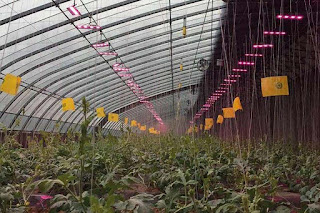In China, scientists are exposing crops to electric fields
Courtesy of Liu Binjang

NewScientist

Inside China's attempt to boost crop yields with electric fields

Read the article. (21 Aug 2019)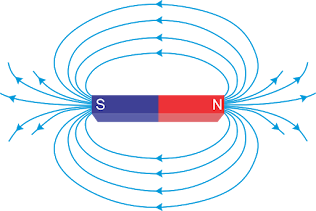Well... it's paramagnetic.

9/25

• magnetism practice problems
• homework - complete the problems above, but omit #35 & 36
• for #33 & 34, the force on a current (wire) equation is:
• F = ILB, where L is length of wire and I is current
• for #34, assume I = 5 amps
• HW answer key - try them before checking the key!

9/27

• explore the Charged Particle in a Magnetic Field simulation -->
• homework - read the rest of Chp 5.4; solve the IB problems on
• magnetism

10/1

• discuss the use of magnetic fields for data storage
• discuss the factors that affect the current in a wire (handout and a few problems)
• Ohm's Law lab
• a few slides on Velocity Selectors and the world's most powerful MRI machine
• homework - practice problems on magnetism; and the handout on current; you should also look at the slides posted above

10/3

• Series and Parallel Circuits lab
• answer key to worksheet with 4 current problems

10/7

• test over fields and forces (electric and magnetic), potential energy, potential, and magnetism in general

10/9

• complete the Series and Parallel Circuits lab
• homework: read in the yellow booklet, skim pp. 8-9 (because it's interesting), read p. 14 and 17-23; finish the lab; test corrections are due 10/17

10/11

• discuss the application of Ohm's Law to series and parallel circuits
• introduce Kirchhoff's laws
• calculate electrical power
• assign Electric Art project - due 10/25
• homework - read/complete pp. 23-31 in the yellow booklet; buy foam board and batteries for the Electric Art project; do test corrections

10/17

10/21

• project work day

10/23

• practice applying Kirchhoff's laws - worksheet (key)
• homework - read Unit 5.3 Electric cells

10/25

• Electric Art project due (both artwork and portfolio)
• discuss potential dividers, diodes and thermistors
• discuss internal resistance of a cell - PowerPoint
• solve IB circuit problems
• homework - complete the packet of circuit problems [key] and the sheet of problems about internal resistance [key]

10/29

• test over circuits
• homework - read Unit 5.3 if you haven't already done so

11/1

• discuss electromagnetic induction (Unit 11.1 in your book)
• Electromagnetic Induction Lab (website, open in Internet Explorer)
• generate a current with a solenoid and magnet
• homework - read Units 5.4 and 11.1 in your textbook (take notes); we'll have a quiz over this material next Friday; also, do test corrections

11/6

• practice using Faraday's law and Lenz's law (notes/problems)
• quiz review - you might find the first 2 videos here helpful
• homework - complete the packet of problems
• homework - read this webpage about induction cooktops, then complete this online reading check (Google form) -- this reading check assignment will be due 11/12, but do it before the test if you have time

11/8

• quiz (test) over electromagnetic induction

11/12 (late start)

11/14

• discuss the workings of a dynamo and DC motor
• discuss measuring current and voltage in an AC circuit - notes with problems
• homework - read the Power Generation booklet and the 2 articles below; complete the packet

11/18

• explore Faraday's law, and the working of a transformer, in the lab
• lab instructions and assistance (the lab report will be graded)
• discuss the role of transformers in ac electricity distribution
• homework - read pp. 444-449 in your textbook; 2nd pd should also do test corrections

11/20

• complete the lab

11/22

• complete IB problems on transformers
• look at a tesla coil
• homework - complete the end-of-chapter problems for Chp 5 (pp. 240-244)

Thanksgiving Break || Thanksgiving Break || Thanksgiving Break

12/3

• analyze a diode bridge rectification circuit
• simple simulation || video with explanation || complex simulation
• homework - finish the Transformers packet, if you haven't already

12/5

• discuss capacitors, including capacitance and the energy stored in a capacitor
• PhET simulation of capacitor

12/9

• analyze the charging and discharging of capacitors in the lab

12/11

• discuss circuits containing capacitors in series and in parallel
• solve IB problems involving capacitors - key

12/13

• quiz over capacitors
• homework - complete the Chps. 10 & 11 end-of-chapter reviews and turn in, along with the Chp. 5 review, by the midterm -- this will serve as a major grade

12/17

• semester review

12/19

• midterm exam

Winter Break​

1/7

• begin Unit 7: Atomic, Nuclear, and Particle Physics
• Unit 7.1: Discrete energy and radioactivity
• atomic spectra and ionizing radiation (worksheet)
• ionizing radiation PowerPoint (we stopped at slide 13)

​[Following Unit 7, we will cover Unit 12, an AHL extension of Unit 7, and then Unit 8. At this point, we will have covered all of the content for the course.]

1/9

• discuss the 3 types of ionizing radiation and when/how they are emitted

1/13

• solve radioactivity problems using the decay constant, activity and half-life
• homework - finish the worksheet

1/15

• solve IB practice problems

1/17

• test over atomic spectra and radioactivity
Last Year's Webpage
Some birds can 'see' magnetic fields.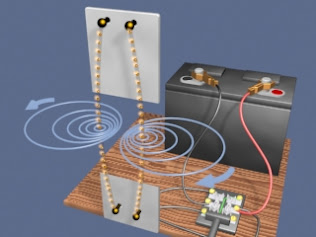B Field around 2 current-carrying wires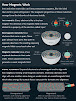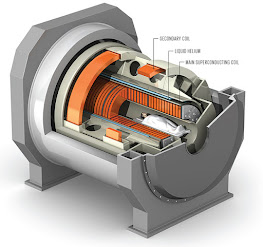prototype MRI machine
A collection of excellent videos explaining electricity, its creation, distribution, manipulation and usage.

Power Generation and Distribution

(Perrone 2016)

View an illustration of Electromagnetic Induction here and also here.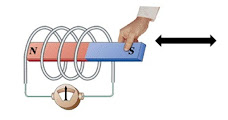These videos are rather advanced, but you may enjoy them.

Explore these interactive tutorials on electricity and magnetism!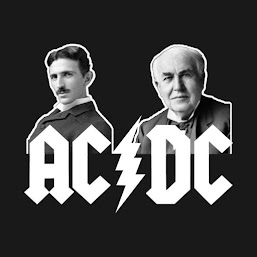Nikola Tesla and Thomas Edison

# Edison's Revenge: Will Direct Current Make a Comeback in the U.S.? - article

Tesla vs Edison: the AC/DC current wars make a comeback - article

Why use 3 phase AC electricity?